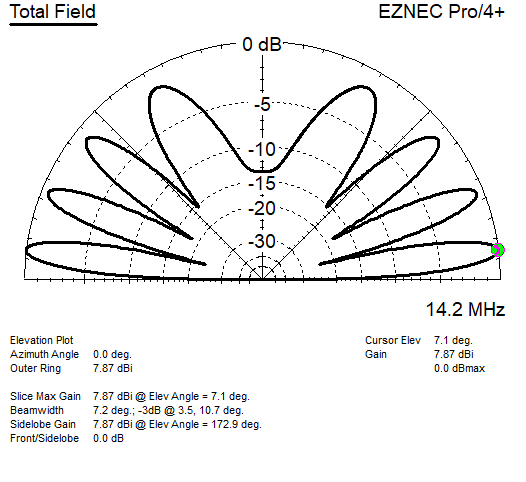### Take off angle of horizontal antenna, vertical max's and min's, as function of antenna height

#### Formulas used:

###### TOA(1.max) = asin(300/F/2/2/H);           1.min = asin(2*300/F/2/2/H) TOA(2.max) = asin(3*300/F/2/2/H);        2.min = asin(4*300/F/2/2/H) etc

Both decimal point and comma are ok.

Give frequency in MHz     λ =

Give antenna height in m

#### Results:

TOA, 1.max =        1. min =

2. max =             2. min =

3. max =             3. min =

4. max =            4. min =

5. max =             5. min =

NaNdeg means the angle is over 90deg.

#### Example, 20m dipole up 42m:Pekka Ketonen 10.8.2022